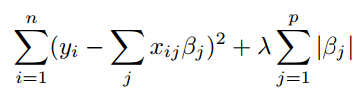### What is Regularization in Machine Learning (Ridge Regression and Lasso Regression)?

Regularization is one of the most important concepts in entire machine learning. It is a technique that discourages learning a more flexible and complex model so as to avoid the risk of overfitting.Y ≈ β0 + β1X1 + β2X2 + …+ ….. βpXp

Here, Y represents the learned relation and beta represents the coefficient estimate for different variables or predictors(X).
The fitting procedure involves a loss function known as the residual sum of squares(RSS). The coefficients are chosen in such a way so that they minimize the loss function.
##### Roles of Regularization:
1. It significantly reduces the variance of the model without a substantial increase in the bias.
2. It is used in the case of Overfitting.
3. It shrinks and regularizes the coefficients for a better prediction, without losing the important properties of the data.

## Ridge Regression:

If there is a noise in the training data than the estimated coefficients will not generalize well in the future, this is where the regularization technique is used to shrink and regularize these learned estimates towards zero.Ridge Regression Optimization Function
Here, lambda is the running parameter that decides how much we want to penalize the flexibility of our model.
If we want to minimize the above function, then these coefficients need to be small. This is how the Ridge Regression technique prevents coefficients from rising too high. It is also called as L2-norm.
##### Case 1: lambda = 0
When the lambda = 0, the penalty term has no effect on the error, and the estimates produced by Ridge Regression will be equal to least square. It means the model will try to minimize the error as much as possible and end up in going the overfitting state i.e., it will be performing well on training data but not on the test or unseen data.
##### Case 2: lambda = Very Large Value
In this case, we will be giving very high weightage to the penalty term, and to reduce the error that is coming from the penalty term the model will learn all the coefficients as zero. So that the error from the penalty may become as less as possible as its weightage is very high. In this scenario, our model’s performance will be pathetic.
##### Case 3: lambda = Reasonable Value
In this scenario, the model will try to minimize the penalty as well as the Residual Sum of Square part and end up learning an optimal set of coefficients by avoiding the overfitting as well as underfitting. In this case, the coefficient’s value will be as less as possible but not equal to zero.

### Lasso Regression:

It is different from Ridge Regression only in terms of the penalty part of the error. In Ridge Regression, we were using the sum of the square of coefficients as the penalty, here we will be using sum of modulus of coefficients as the penalty term, it is also called L1-norm.Lasso Regression Optimization Function
The Lasso can be thought of as an equation where the summation of modulus of coefficients is less than or equal to s. Here, s is a constant that exists for each value of shrinkage factor λ. These equations are also referred to as constraint functions.

NOTE: we can analyze lambda similarly as explained in the case of Ridge Regression.

One of the drawbacks with lasso regression is that it creates the sparsity among the coefficients means more number of coefficients are going to be zero. Its reason is that here we are directly using the absolute sum of coefficients as the penalty. So to reduce the error as less as possible it eliminates the less important features and makes their coefficients as zero.

## Ridge vs Lasso Regression:

Lasso Regression is also used to find the important variables in the dataset. Lasso Regression will shrink the coefficients towards 0 indicating these variables do not contribute to output variable much in order to develop sparse models.

On the other hand, Ridge Regression will shrink the coefficients for the least important predictors very close to 0, but it will never make them 0 ie in the final model all the variables will be present.